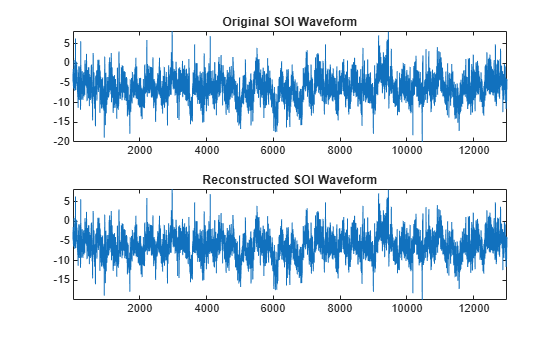# imodwpt

Inverse maximal overlap discrete wavelet packet transform

## Syntax

``xrec = imodwpt(coefs)``
``xrec = imodwpt(coefs,wname)``
``xrec = imodwpt(coefs,lo,hi)``

## Description

example

````xrec = imodwpt(coefs)` returns the inverse maximal overlap discrete wavelet packet transform (inverse MODWPT), in `xrec`. The inverse transform is for the terminal node coefficient matrix (`coefs`) obtained using `modwpt` with the default length 18 Fejér-Korovkin (`"fk18"`) wavelet.```

example

````xrec = imodwpt(coefs,wname)` returns the inverse MODWPT using the wavelet specified by `wname`.```

example

````xrec = imodwpt(coefs,lo,hi)` returns the inverse MODWPT using the orthogonal scaling filter, `lo`, and wavelet filter, `hi`.```

## Examples

collapse all

Obtain the MODWPT of an ECG waveform and demonstrate perfect reconstruction using the inverse MODWPT.

```load wecg; wpt = modwpt(wecg); xrec = imodwpt(wpt); subplot(2,1,1) plot(wecg); title('Original ECG Waveform'); subplot(2,1,2) plot(xrec); title('Reconstructed ECG Waveform');```Find the largest absolute difference between the original signal and the reconstruction. The difference is on the order of $1{0}^{-11}$, which demonstrates perfect reconstruction.

`max(abs(wecg-xrec'))`
```ans = 1.7903e-11 ```

Obtain the MODWPT of Southern Oscillation Index data using the Daubechies extremal phase wavelet with two vanishing moments (`'db2'`). Reconstruct the signal using the inverse MODWPT.

```load soi; wsoi = modwpt(soi,'db2'); xrec = imodwpt(wsoi,'db2');```

Obtain the MODWPT of Southern Oscillation Index data using specified scaling and wavelets filters with the Daubechies extremal phase wavelet with two vanishing moments (`'db2'`).

```load soi; [lo,hi] = wfilters('db2'); wpt = modwpt(soi,lo,hi); xrec = imodwpt(wpt,lo,hi);```

Plot the original SOI waveform and the reconstructed waveform.

```subplot(2,1,1) plot(soi) title('Original SOI Waveform'); subplot(2,1,2) plot(xrec) title('Reconstructed SOI Waveform')```## Input Arguments

collapse all

Terminal node coefficients of a wavelet packet tree, specified as a matrix. You must obtain the coefficient matrix from `modwpt` using the `'FullTree',false` option. `'FullTree',false` is the default value of `modwpt`.

Data Types: `single` | `double`

Synthesizing wavelet used to invert the MODWPT, specified as a character vector or string scalar. `wname` must be the same wavelet used in the analysis with `modwpt`.

Filters, specified as a pair of even-length real-valued vectors. `lo` is the orthogonal scaling filter and `hi` is the orthogonal wavelet filter. `lo` and `hi` must be the same filter pair used in the analysis with `modwpt`. You cannot specify both `wname` and a scaling-wavelet filter pair.

Data Types: `single` | `double`

## Output Arguments

collapse all

Inverse maximal overlap discrete wavelet packet transform, returned as a row vector. The inverse transform is the reconstructed version of the original signal based on the MODWPT terminal node coefficients. `xrec` has the same number of columns as the input `coefs` matrix.

 Percival, Donald B., and Andrew T. Walden. Wavelet Methods for Time Series Analysis. Cambridge Series in Statistical and Probabilistic Mathematics. Cambridge ; New York: Cambridge University Press, 2000.

 Walden, A. T., and A. Contreras Cristan. “The Phase–Corrected Undecimated Discrete Wavelet Packet Transform and Its Application to Interpreting the Timing of Events.” Proceedings of the Royal Society of London. Series A: Mathematical, Physical and Engineering Sciences 454, no. 1976 (August 8, 1998): 2243–66. https://doi.org/10.1098/rspa.1998.0257.